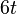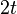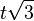# Hexagon

In geometry, a hexagon is a polygon with six edges and six vertices. A regular hexagon has the Schläfli symbol {6}.

## Regular hexagon

The internal angles of a regular hexagon (one where all sides and all angles are equal) are all 120° and the hexagon has 720 degrees. It has six lines of symmetry. Like squares and equilateral triangles, regular hexagons fit together without any gaps to tile the plane (three hexagons meeting at every vertex), and so are useful for constructing tessellations.

The cells of a beehive honeycomb are hexagonal for this reason and because the shape makes efficient use of space and building materials. The Voronoi diagram of a regular triangular lattice is the honeycomb tessellation of hexagons.

The area of a regular hexagon of side length$t\,\!$ is given by$A = \frac{3 \sqrt{3}}{2}t^2 \simeq 2.598076211 t^2.$

The perimeter of a regular hexagon of side length$t\,\!$ is, of course,$6t\,\!$, its maximal diameter$2t\,\!$, and its minimal diameter$t\sqrt{3}\,\!$.

There is no platonic solid made of regular hexagons. The archimedean solids with some hexagonal faces are the truncated tetrahedron, truncated octahedron, truncated icosahedron (of soccer ball and fullerene fame), truncated cuboctahedron and the truncated icosidodecahedron.